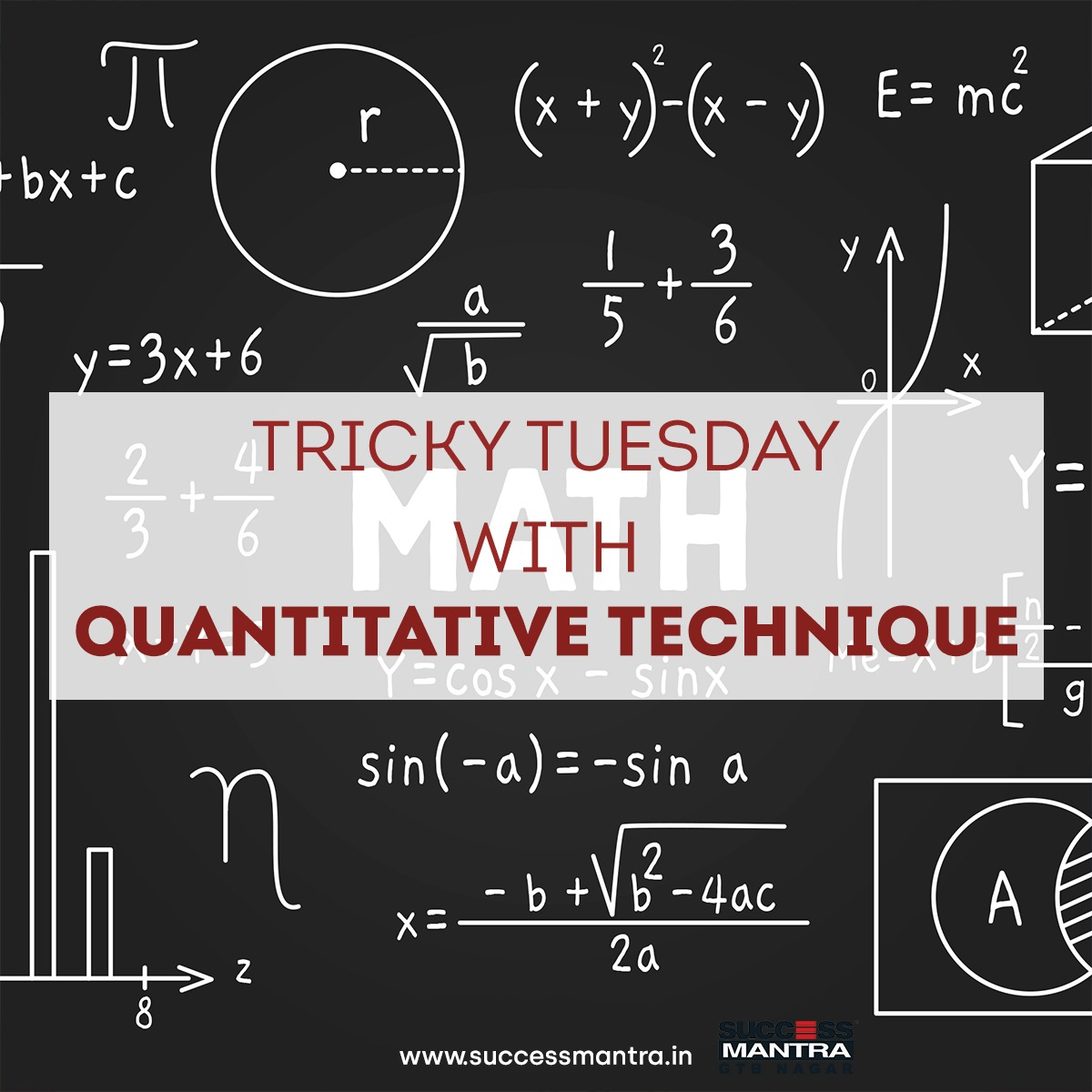## Questions On Quantitative Techniques SMQTQ0381. Present average of age of A and B is 6x – 15 years. Present average age of A, B and C is 4x + 6 years. If present age of B is 25% less than the present age of C and 20% more than the present age of A, then find the present age of A.

A. 30 years
B. 33 years
C. 36 years
D. 39 years

2. The age of Arjun is (x + 5) years. The ratio of the age of Arjun 2 years hence to the age of Draupadi 1 year before is 6 : 5 respectively. If Arjun is 4 years older than Draupadi, then find the age of Shubhadra whose age is 4 years less than the average age of Arjun and Draupadi taken together.

A. 30 years
B. 36 years
C. 34 years
D. 32 years

3. Arun and Meena recently celebrated their golden anniversary and their daughter Seema’s birthday. If the age of Seema 18 years after her parents’ marriage and her age at their golden anniversary is in the ratio 5 : 21, how many years after the marriage was Seema born?

A. 8 years
B. 15 years
C. 12 years
D. 10 years

4. Seven years later from now A will be as old as B was 4 years ago. C was born 2 years ago. The average age of A, B and C 10 years later will be 33yrs. What is the present age of A?

A. 30 years
B. 28 years
C.31 years
D. 29 years

5. P’s age 8 years ago is equal to the sum of the present ages of his son and his daughter. 5 years hence, the ratio between his daughter’s age and his son’s age will be 7 : 6 respectively. P’s wife is 7 years elder than him. His wife’s present age is thrice the present age of his son. What is his daughter’s present age?

A. 18 years
B. 20 years
C. 23 years
D. 28 years

6. The present average age of a group of 60 athletes is 50% more than the average age of the 60 athletes 5 years ago. The average age of the group will become Y, if two new athletes of 22 year and 38 years join the group. Find the value of Y.

A. 14.44
B. 17.54
C. 15.48
D. 16

7. The ratio between the present ages of Amon and Chan is 3 : 4. The ratio of Bevan’s age after 5 years to Chan’s age 1 year ago is 4 : 3 and four times the difference in ages of Chan and Amon is one more than the age of Bevan. Find the average of the present ages of Amon and Chan.

A. 20 years
B. B. 10 years
C. 14 years
D. 15 years